# Geometric Shapes List with Names and Drawings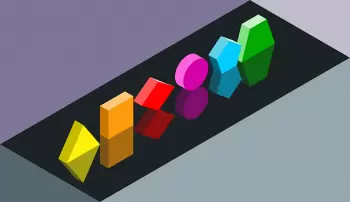Flat geometric shapes are spaces delimited by flat surfaces. These elements are composed of straight and curved lines connected, for example, the circle, the square, or the triangle. Polygons are those types of geometric shapes that are bounded by straight lines.

In contrast, solid shapes are the shapes represented in a three-dimensional space. These 3d shapes occupy a volume in space. An example of a solid shape is the cube, which is a shape that has 12 edges, eight vertices, and six faces.

The branch of mathematics that studies geometric shapes is geometry.

## Geometric Shape Names Table

Here is a basic list of 2D shapes with their name, a drawing, and a brief description, of the main named regular geometric figures:

 Circle The circle is made up of a single circular segment. It is a circular sector in which the ends are connected. Also, all points on this curved line are the same distance from a center point.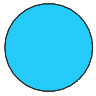Ellipse The ellipse is a geometric figure formed by a curved line with centers called foci. The main characteristic of the ellipse is that the sum of the distance from a point to one of the foci with the distance from the same point to another of the foci is always constant.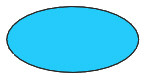Triangle The triangle is the simplest polygon that can be represented. It comprises three sides, three vertices, and three angles.Depending on their angles, there are three types of triangles: equilateral, isosceles, and scalene.Equilateral triangle: It has three equal sides.Isosceles triangle: It only has two equal sides.Scalene triangle: The three sides of the triangle are different.This shape is fascinating because all polygons can be split into triangles. Since it is effortless to calculate the triangle's area, it is an interesting way to calculate any polygon's area.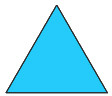Square The square is a quadrilateral formed by four sides with equal length and equal interior angles (90 degrees).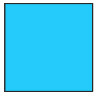Rectangle The rectangle is a geometric shape formed by four edges. Sides are equal two by two: sides of the same length are parallel. Like the square, the angle formed by the edges that touch is 90 degrees.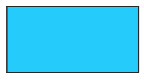Diamond A rhombus is a quadrilateral in which all four sides are equal, and the angles are equal two by two.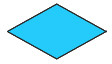Rhomboid A rhomboid is a quadrilateral with two pairs of equal sides. Its angles are also equal in pairs.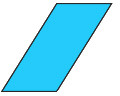Trapeze Trapezoid is a polygon with four sides of which two of the opposite sides are parallel.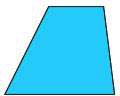Trapezoid Trapezoid is a quadrilateral polygon in which none of the opposing sides is parallel.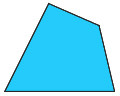Pentagon A pentagon is a polygon with five corners. A regular pentagon is a pentagon where all sides and angles are equal.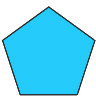Hexagon A pentagon is a polygon with six sides, angles, and vertices.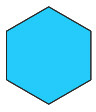## What Are Curved Shapes?

The curved geometric shapes are the figures that can be represented on a flat surface in which at least one of its sides is a curved line.

The curved shapes are the circle and the ellipse in the list of shapes above. In both cases, we are dealing with closed curved lines.

## What Is a Polygon?

In geometry, polygons are two-dimensional geometric shapes composed of a finite sequence of consecutive straight line segments enclosing a region in the plane.  These segments are called sides, and the intersect points are called vertices. The polygon is the two- dimensional case of the polytope.

The segments are called edges or sides, and the ends of the sides are called vertices or corners of the polygon.

A polygon is crossed if at least two nonconsecutive sides intersect and simple if the intersection of two sides is empty or reduced to a vertex for two consecutive sides. The sum of the angles of a simple polygon ( convex or not) depends only on its number of vertices.

In the case of simple polygons, the polygon and its interior are often confused by calling the surface bounded by the closed polygonal line a polygon.